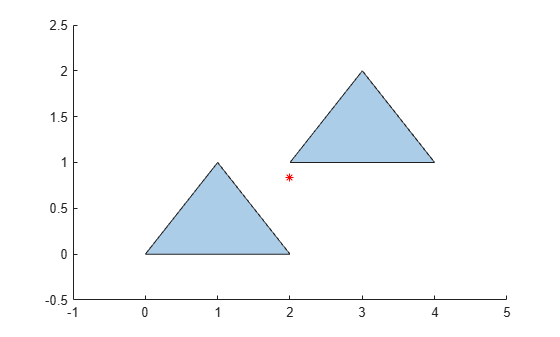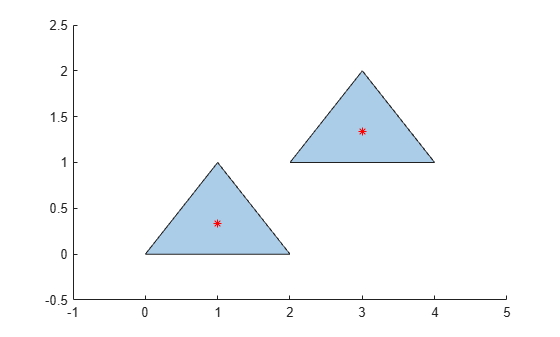# centroid

Centroid of `polyshape`

## Syntax

``[x,y] = centroid(polyin)``
``[x,y] = centroid(polyin,I)``

## Description

example

````[x,y] = centroid(polyin)` returns the x-coordinates and the y-coordinates of the centroid of a `polyshape`.```

example

````[x,y] = centroid(polyin,I)` returns the coordinates of the centroid of the `I`th boundary of `polyin`.This syntax is only supported when `polyin` is a scalar `polyshape` object.```

## Examples

collapse all

Compute the centroids associated with a multiregion polygon.

Create a polygon containing two solid regions, and then compute its centroid.

```x1 = [0 1 2]; y1 = [0 1 0]; x2 = [2 3 4]; y2 = [1 2 1]; polyin = polyshape({x1,x2},{y1,y2}); [x,y] = centroid(polyin); plot(polyin) hold on plot(x,y,'r*') hold off```To compute the centroid of each region separately, specify the boundary indices of each region in the second argument.

```[x,y] = centroid(polyin,[1 2]); plot(polyin) hold on plot(x(1),y(1),'r*',x(2),y(2),'r*') hold off```Alternatively, you can compute the centroid of each region by first separating the regions into separate `polyshape` objects. Use the `regions` function to create an array of `polyshape` objects, where each element of the array is a `polyshape` defining one of the triangular regions.

`polyarray = regions(polyin)`
```polyarray = 2x1 polyshape array with properties: Vertices NumRegions NumHoles ```
`[x,y] = centroid(polyarray)`
```x = 2×1 1 3 ```
```y = 2×1 0.3333 1.3333 ```

## Input Arguments

collapse all

Input `polyshape`, specified as a scalar, vector, matrix, or multidimensional array.

Data Types: `polyshape`

Boundary index, specified as a scalar integer or vector of integers. Each element of `I` corresponds to a single boundary of the input `polyshape`.

Data Types: `double` | `single` | `int8` | `int16` | `int32` | `int64` | `uint8` | `uint16` | `uint32` | `uint64`

## Output Arguments

collapse all

x-coordinate of the centroid of a `polyshape`, returned as a scalar, vector, matrix, or multidimensional array. If the input is an array of `polyshape` objects, then `x` contains the x-coordinates of the centroid of each `polyshape`.

y-coordinate of the centroid of a `polyshape`, returned as a scalar, vector, matrix, or multidimensional array. If the input is an array of `polyshape` objects, then `y` contains the y-coordinates of the centroid of each `polyshape`.

## Version History

Introduced in R2017b